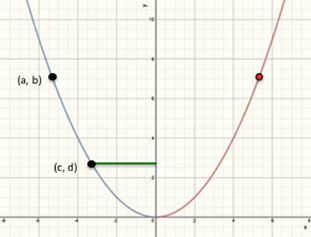# Parabolas Lesson 5 Episode 3 (Teachers)

### Reflecting

Keoni and Sasha compare the equations for two parabolas: y = x2/8 and y = x2/4. They make two different conjectures about the equation for a parabola with a focus at 3 units above the vertex.

### Episode SupportsStudents’ Conceptual Challenges

With only two equations, differing patterns can emerge that might not work in the general case.

➤ Sasha and Keoni offer two different conjectures for the equation of a third parabola: y = x2/12 and y = x2/16 [1:16-1:45].  They resolve their differences in Episode 4.

Focus Questions

For use in a classroom, pause the video and ask these questions:

1. [Pause video at 0:57]. Take a moment to examine the focus and directrix that Sasha just drew. When the vertex of the parabola is on the origin, how are the positions of the focus and directrix related?

2. [Pause video at 1:09]. What are your conjectures about the equation for the third parabola?

Supporting Dialogue

Provide opportunities for productive disagreement by asking:

1. Keoni and Sasha have two conjectures. Who thinks the next parabola will have an equations of y = x2/16? Who thinks the equation will be y = x2/12?

2. Can we have one person to defend the first conjecture? What about someone for the other? Let’s hear each case.

Math Extensions

1. The graph below represents the equation y = x2/4 . Consider the part of the graph colored blue. Label some coordinates of points on the blue part of the graph. What is common about the coordinates of every point on the blue part of the graph?

2. Notice the point (a, b) on the graph. Represent the coordinates of the red point using the a and the b.  Explain your thinking.

3. Notice the line segment shown in green. In mathematics, it is a convention to represent distances with positive numbers. Coordinates can be negative, but distances and lengths must be positive. How can you use the coordinates to represent the length of the green line, which is the distance from the blue parabola to the y-axis?